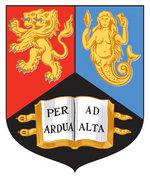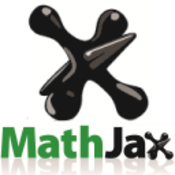MathJax, SRE and some Diagrams

# MathJax, SRE and some Diagrams Technical Background

University of Birmingham, UKcs.bham.ac.uk/~vxs

MathJax Consortiummathjax.org

Progressive Accessiblity Solutionsprogacc.com

Indian Instiute of Technology, Delhiiitd.ac.in

## Background

• Raised mathematics project
• Automatically translate mathematical literature into tactile formats
• Mainly aimed at advanced math textbooks and articles
• Project is a mix of reseach and novel technical development

## What is MathJax?

• MathJax is an open-source JavaScript library for rendering Mathematics in all browsers
• Can take LaTeX, AsciiMath, and MathML as input
• Generates browser output, e.g. HTML/CSS, SVG
• MathJax is the de facto rendering solution of most Mathematics on the web
• Standard Maths rendering solution for many publishers
• Around for over 10 years, lastest version 3.2.2 released a couple of weeks ago

In our context: translation of LaTeX to Nemeth Braille

## Speech Rule Engine

• Javascript library for translating Math into Speech available at github
• Only Math speech solution in JavaScript: Runs in browser, command line, as node module.
• Speech solution for: ChromeVox, MathJax, EquatIO, MathLive, \ldots
• Based on semantic interpretations via pattern matching techniques
• Ignores any poor syntax (or at least tries to)
• Math markup (e.g., LaTeX, MathML) are not very expressive, sometimes bloated

## SRE in MathJax

$$x = {-b \pm \sqrt{b^2-4ac} \over 2a}$$

• Accessibility extension pulls in SRE
• Speech (or Braille) with
• Interactive Exploration
• Highlighting, magnification, etc.
• All this is done using a semantic intepretation

## Generate Semantics

    ax^2+bx+c=0

$<mi>a</mi> <msup> <mi>x</mi> <mn>2</mn> </msup> <mo>+</mo> <mi>b</mi> <mi>x</mi> <mo>+</mo> <mi>c</mi> <mo>=</mo> <mn>0</mn>$


## Nemeth in SRE

• Once semantics is embedded speech and other alternative formats can be easily generated
• Tactile output, Abstraction, Summaries $$\vec{\nabla} \times \vec{F} = \left( \frac{\partial F_z}{\partial y} - \frac{\partial F_y}{\partial z} \right) \mathbf{i} + \left( \frac{\partial F_x}{\partial z} - \frac{\partial F_z}{\partial x} \right) \mathbf{j} + \left( \frac{\partial F_y}{\partial x} - \frac{\partial F_x}{\partial y} \right) \mathbf{k}$$
Click or focus on the formula and press ENTER to start, Escape to quit.

## MathJax on the Server

• work on the server as easily as in the browser
• works in non-standard DOMs (e.g., markdown or PreTeXt)
• separable pipeline for
• finding
• converting
• annotating
• rendering math
• E.g., LaTeX in a document can be translated into SVG with speech or Braille annotation.

## 2D in Nemeth

• Nemeth for embossing requires
• Large fractions
• Matrices, vectors
• Equation systems
• Cayley tables
• SRE can generate 2D output
• Uses same rules as for Braille display output during screen reading
• Applies abstract styling rules
• Some sample output

## Quality Assurance

• We developed a Webapp for Nemeth Translation
• Basic idea:
• Proof read and correct automatically translated math
• Easy reference to context via links source
• Selection of semantically unique samples vs full list of expressions

## Some Challenging Examples

Nemeth is challenging to get right automatically, but sometimes automation is better:

• Subtlety of spaces:
• $44,352,000$ is a single number and transcribes as ⠼⠲⠲⠒⠢⠆⠴⠴⠴
• $(0110,1110,0110)$ is not a single number but a vector, hence ⠷⠼⠴⠂⠂⠴⠀⠼⠂⠂⠂⠴⠀⠼⠴⠂⠂⠴⠾
• $(n, E) = (451{,}231)$ The right hand side is not a single number ⠷⠝⠠⠀⠠⠑⠾⠀⠨⠅⠀⠷⠲⠢⠂⠠⠀⠆⠒⠂⠾
• Context helps $$PAP^{-1} = B$$ is not an abbreviation: ⠠⠏⠠⠁⠠⠏⠘⠤⠂⠀⠨⠅⠀⠠⠃

• Automated generation of tactile graphics is challenging
• Generated with LaTeX packages
• Many Standard techniques do not work